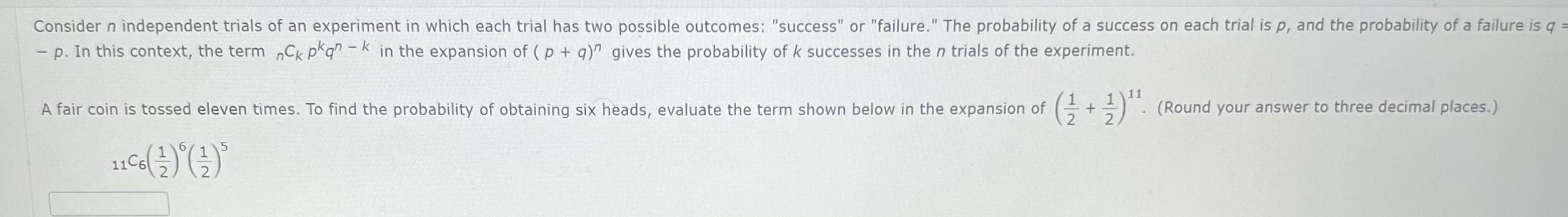Question:

# Consider n independent trials of an experiment in which each

Last updated: 11/20/2023Consider n independent trials of an experiment in which each trial has two possible outcomes success or failure The probability of a success on each trial is p and the probability of a failure is q p In this context the term nCk pkqnk in the expansion of p q gives the probability of k successes in the n trials of the experiment A fair coin is tossed eleven times To find the probability of obtaining six heads evaluate the term shown below in the expansion of Round your answer to three decimal places 11 11 6 5